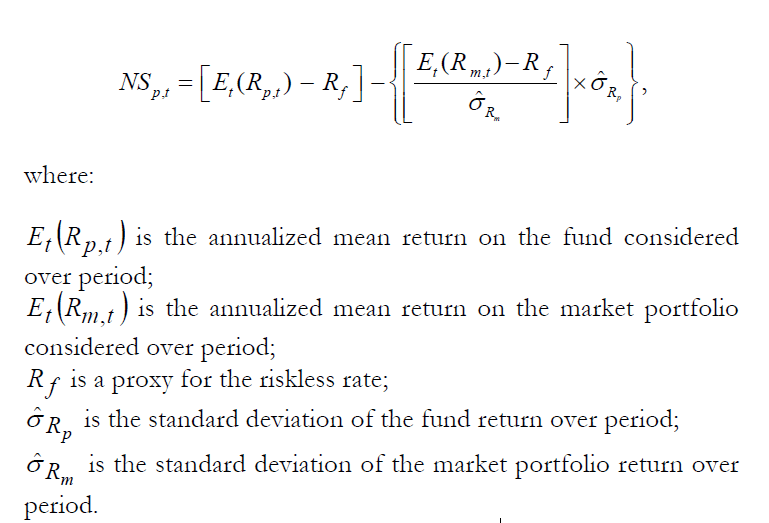# Performance Evaluation Managed Portfolio: Jensen’s Alpha, Fama’s Net Selectivity Ratio

Jensen’s alpha (Jensen, 1968) is based on the Capital Asset Pricing Model (CAPM) of Sharpe (1964), Lintner (1965), and Mossin (1966). The alpha represents the amount by which the average return of the portfolio deviates from the expected return given by the CAPM. The CAPM specifies the expected return in terms of the risk-free rate, systematic risk, and the market risk premium. The alpha can be greater than, less than, or equal to zero. An alpha greater than zero suggests that the portfolio earned a rate of return in excess of the expected return of the portfolio. Jensen’s alpha is given by:where a is the Jensen’s alpha, rp the return of the portfolio, rm the return of the market portfolio, rf the risk-free rate, and bp the beta of the portfolio.

Using the same set of numbers from the previous example, the alpha of the managed portfolio and the market portfolio can be computed as follows. The expected return of the managed portfolio is 4 percent + 1.5 (13 percent — 4 percent) = 17.5 percent. Therefore, the alpha of the managed portfolio is equal to the actual return less the expected return, which is 20 percent — 17.5 percent = 2.5 percent. Since we are measuring the expected return as a function of the beta and the market risk premium, the alpha for the market is always zero. Thus, the managed portfolio has earned a 2.5 percent return above that must be earned given its market risk. In short, the portfolio has a positive alpha, suggesting superior performance.

When the portfolio is well diversified all three methods Sharpe, Treynor, and Jensen – will give the same ranking of performance. In the example, the managed portfolio outperformed the market on the basis of all three ratios. When the portfolio is not well diversified or when it represents the total wealth of the investor, the appropriate measure of risk is the standard deviation of returns of the portfolio, and hence the Sharpe ratio is the most suitable. When the portfolio is well diversified, however, a part of the total risk has been diversified away and the systematic risk is the most appropriate risk metric. Both Treynor ratio and Jensen’s alpha can be used to assess the performance of well-diversified portfolios of securities. These two ratios are also appropriate when the portfolio represents a sub-portfolio or only a part of the client’s portfolio. Chen (1981, 1986) examined the statistical distribution of Sharpe, Treynor, and Jensen measures and show that the empirical relationship between these measures and their risk proxies is dependent on the sample size, the investment horizon and market conditions. Cumby and Glen (1990), Grinblatt and Titman (1994), Kallaberg et al. (2000), and Sharpe (1998) have provided evidence of the application of performance evaluation techniques.

Description of the Measure:

The Fama’s Net Selectivity Measure is an absolute measure of performance. It is given by the annualized return of the fund, deducted the yield of an investment without risk, minus the standardized expected market premium times the total risk of the portfolio under review.

Interpretation:

The Fama’s Index gives the excess return obtained by the manager that cannot have been obtained investing in the market portfolio.

It compares the extra return obtained by the portfolio manager with a specific risk and the extra return that could have been obtained with the same amount of systematic risk.

Use:

The magnitude of the Ferson-Schadt Measure depends on three variables: the return of the fund, the market return and the manager’s stock picking ability.

Potential Misuse:

Accuracy and reliability of this measure is based on the quality of the market proxy.

Formula:Two year data of weekly series is considered.

## One thought on “Performance Evaluation Managed Portfolio: Jensen’s Alpha, Fama’s Net Selectivity Ratio”

error: Content is protected !!